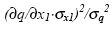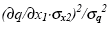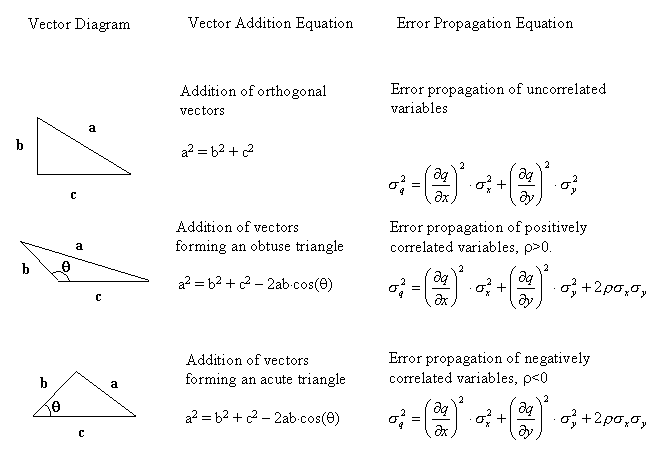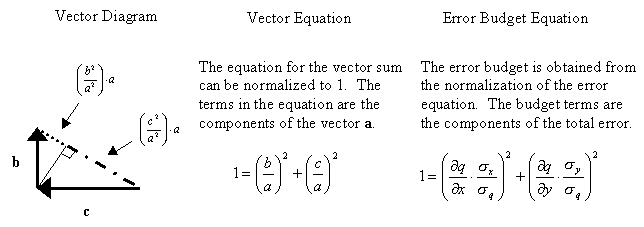### Ernest Lo. 2005. Gaussian error propagation applied to ecological data: post-ice-storm-downed woody biomass. Ecological Monographs 75:451–466.

Appendix B. Geometrical analogies of the error propagation and error budget equations, with the addition of vectors.

The propagation of errors has a direct geometrical analogy with the addition of vectors.

There is an exact correspondence between the vector quantities and the error terms, which is described pictorially and mathematically in Table B1. This geometrical analogy provides a helpful physical picture for the mechanism of error propagation.

The calculation of error for uncorrelated variables represented by Eq.1 can be thought of as an addition of orthogonal vectors. The squared error is analogous to the vector sum or hypotenuse, while the input variable contributions are analogous to the component vectors, as shown in Table B1, row 1. Equation 1 is thus analogous to the well-known Pythagorean Theorem.

This analogy can be extended to the case of correlated variables. The addition of non-orthogonal vectors is described by the cosine law (an extended form of the Pythagorean Theorem), which is mathematically analogous to Eq. 2. The cosine term in the cosine law represents the contribution produced by the correlation term; the cosine itself ranges from -1 to +1 and is analogous to the correlation coefficient ρ . Table B1, row 2 illustrates the case of positive correlation — the geometrical analogy to positive correlation is an obtuse opposite angle θ which enhances the magnitude of error represented by the vector a. Table B1 row 3 describes the case of negative correlation — the effect of the negative correlation is represented by an acute angle θ, which reduces the magnitude of the error represented by the vector a.

The equation for the error budget can also be pictured geometrically. The various percentage contributions to the total error in the budget are mathematically analogous to the projections of their vectors onto the hypotenuse, which represents the total error. This geometric analogy is depicted in Table B2. The total error represented by the length of the vector a is composed of two fractions, (b/a)2 and (c/a)2. These latter terms are analogous to the budget termsandfrom Eq. 6.

TABLE B1. The analogy between vector addition and error propagation is shown; the magnitude of vector a represents the total error and it is produced by the sum of vectors b and c which represent the input errors.TABLE B2. The vector a is composed of two contributions from the vectors b and c; similarly, the error budget is composed of analogous contributions from the input errors.[Back to M075-023]